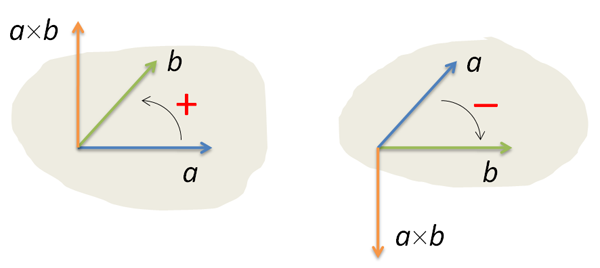# A Point Localization in a Polygon

AlgorithmsI have recently come across a post dedicated to solving the task of locating a point in a polygon: there is a polygon (a closed broken line with no self-intersections) and we are to determine, whether the given A point is inside the polygon or not. You may wonder, how such purely mathematic task can be related to the theory of algorithms. But it actually can. Localization is a classic task in computational geometry (do not confuse with computer graphics). To begin with, look at the picture on the right that depicts a polygon of Peano curvetype . Try to tell, whether the red point is inside or outside the polygon. We are going to review a simple variation of the given task, when the given polygon is convex.

It is clear that if a polygon is a triangle or a quadrangle, there will be no full-on algorithm, but instead there will be three or four formulas to implement. But if there are a million or one hundred million vertices in a polygon, such task will be quite algorithmic. A simple algorithm (starting a beam from the given point and calculating its intersections with the polygon sides) is linear with regard to n number of polygon vertices, since we should check the beam intersection with all sides of the polygon. But can we do it faster? Is there any sublinear algorithm to solve the localization task in the given statement? And the answer is: “Yes”. We can solve this task during O(log2n) time. You will find more details of the base case, when a polygon is not required to be convex, in a great book . Now, we are going to look at the way we can apply the binary search algorithm to localization task in a convex polygon.

## Some Theory

Let there be A, B and C points given. Suppose we look from point A to point B. Where will C point be in this case – on the left or on the right from our direction? We can answer this question with the help of a=AB and b=BC vectors multiplication, or rather with the help of z-coordinate of such multiplication.

We can calculate it via a simple formula: z=axby-aybx. If z>0, the sought rotation is left, but if z<0 – the rotation is right. If z=0, three points lie along one straight line (a and b vectors are said to be collinear).The code in Python, calculating rotate direction, is primitive (the points are represented by lists of length 2):

``````def rotate(A,B,C):
return (B-A)*(C-B)-(B-A)*(C-B)
``````

We can do a lot of useful things with the help of this function. For example, we can determine, whether AB and CD given segments intersect. By the way, it’s another base operation in computational geometry. We are going to need it soon (just once). The idea is simple. Two segments intersect only when the ends of one segment are on different sides from the other one, and vice versa.To check, whether C and D points are on different sides from AB segment, we should look at rotate(A,B,C) and rotate(A,B,D) directions. If the signs of these expressions are different, AB intersects CD segment (in the internal point). The signs of two numbers are different only if their multiplication is negative. Therefore, the criterion of AB and CD intersection is negativeness of rotate(A,B,C)*rotate(A,B,D) and rotate(C,D,A)*rotate(C,D,B) expressions. If we replace strict negativeness with nonpositiveness, we will also find intersections in end points of segments. What we are going to need is some intermediate variant, when one multiplication is nonstrictly compared with null and the other one is strictly compared:

``````def intersect(A,B,C,D):
return rotate(A,B,C)*rotate(A,B,D)<=0 and rotate(C,D,A)*rotate(C,D,B)<0
``````

The reason of such a strange choice of inequalities is peculiarity of future application of the given function (in particular, A, B and C points will be different vertices of a convex polygon, while D will be the point we are trying to localize).

Now let’s move on to localization itself. There’s P convex polygon consisting of n vertices and an A point. Suppose that vertices in P are numerated counterclockwise (bypass direction along P perimeter is said to be positive).According to the idea of an algorithm, we will take the first vertex of P polygon and try to define, to what PiP0Pi+1 segment (angle) A point will get to. First of all, let’s check that A gets to Pn-1P0P1 segment. If it does not, A is definitely outside the polygon.The code:

``````def pointloc(P,A):
n = len(P)
if rotate(P,P,A)<0 or rotate(P,P[n-1],A)>0:
return False
``````

Then do everything like in a classic binary search. Suppose that p=1, r=n-1 (the boundaries of the current segment), calculate the average vertex q=(p+r)/2. Look where A is located with regard to P0Pq vector. If it is on the left, replace r with q. If it’s on the right, replace p with q.

Go on doing so until r-p=1:

``````p, r = 1, n-1
while r-p>1:
q = (p+r)/2
if rotate(P,P[q],A)<0: r = q
else: p = q
``````

Now, we should check, whether P0A и PpPr segments intersect.

If they do, A point is outside the polygon. If they do not intersect, it’s inside.The code:

`return not intersect(P,A,P[p],P[r])` That’s pretty much it…

It’s no brainer that algorithm complexity is the same of a classical binary search algorithm – O(log2n). But this algorithm is meant for convex polygons. The considered above algorithm operates with several nonconvex polygons but of quite a specific type. All diagonals leading from the null vertex should lie inside the polygon:The multipurpose localization algorithm also operates on the principle of binary search, but it’s much more complicated inside.